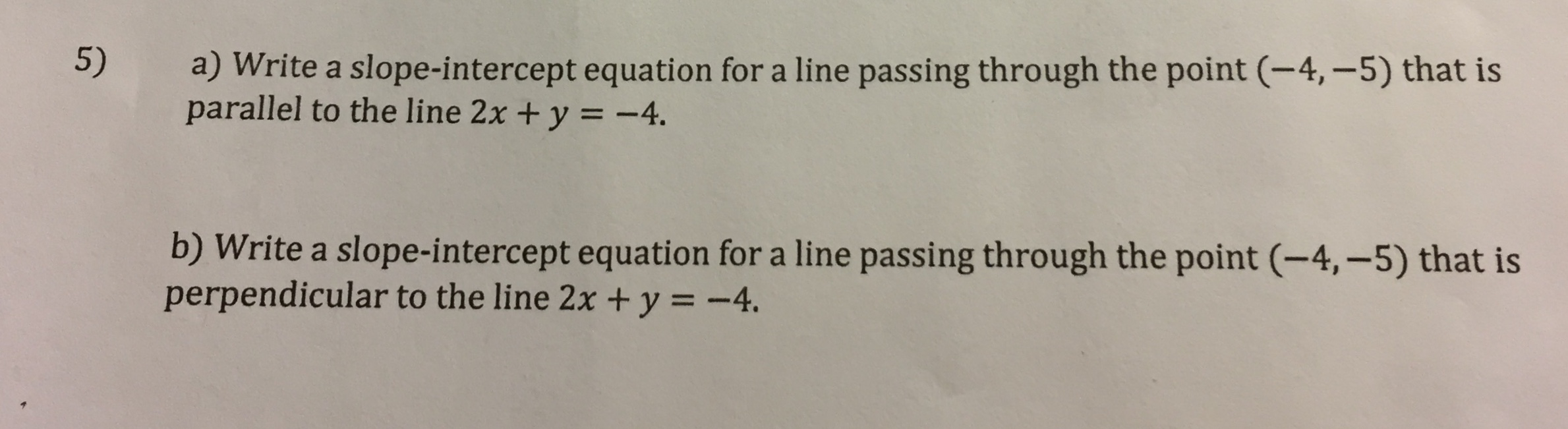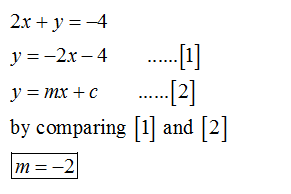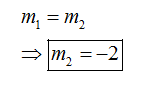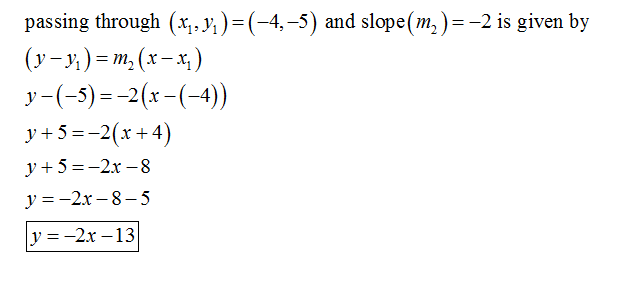# 5)a) Write a slope-intercept equation for a line passing through the point (-4,-5) that isparallel to the line 2x +y = -4.b) Write a slope-intercept equation for a line passing through the point (-4,-5) that isperpendicular to the line 2x + y = -4.

Question
1 viewshelp_outlineImage Transcriptionclose5) a) Write a slope-intercept equation for a line passing through the point (-4,-5) that is parallel to the line 2x +y = -4. b) Write a slope-intercept equation for a line passing through the point (-4,-5) that is perpendicular to the line 2x + y = -4. fullscreen
check_circle

star
star
star
star
star
1 Rating
Step 1

Given:

Equation of a line is 2x+y=-4

Write this equation in the form of y = m x + c. So, the equation becomes:Step 2

Part (a)   We know the condition of the parallel line:Step 3

Now, find the equation of the line:...

### Want to see the full answer?

See Solution

#### Want to see this answer and more?

Solutions are written by subject experts who are available 24/7. Questions are typically answered within 1 hour.*

See Solution
*Response times may vary by subject and question.
Tagged in

### Algebra